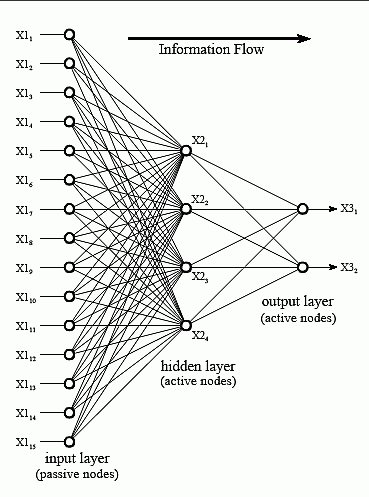2 views

I have a 20 sample of one thing that I want to use as an input in an Artificial neural network, my data is 1-Coordination (pairs of x, y) 2-Sediment size(m). The data should be normalized? Also, what about the efficiency of the network, with this amount of data, Does it work?? If not, What is your suggestion?

by (108k points)

Depending upon the complexity of the model, how many datasets can have determined the number of inputs. The nodes of the input layer are passive, meaning they do not modify the data instead they just receive a single value on their input and duplicate the value to their multiple outputs.This is the most common structure of the neural networks. The input layer nodes are passive, relaying the single input to multiple outputs. Here is a code for output accepting input values:

y = f(w_1*x_1 + w_2*x_2 + c)

where f stands for ‘a function of’, x_n is the n-th input, w_n is the n-th weight of that input, and c is the constant for the node with output y.

First, you will have to normalize the inputs using the range (or maximum) value for each input. For example, to normalize the month inputs, we could use the maximum value '12' to divide all months input value.

Become a master of Artificial Intelligence by going through this online ai course!